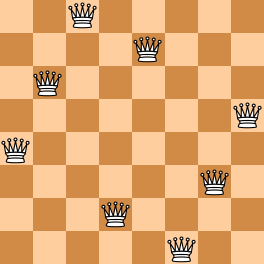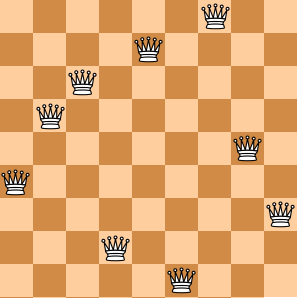The "eight queens puzzle" is the problem of placing eight chess queens on an 8×8 chessboard so that no two queens threaten each other. Thus, a solution requires that no two queens share the same row, column, or diagonal. The eight queens puzzle is an example of the more general N queens problem of placing N non-attacking queens on an N×N chessboard. (From Wikipedia - "Eight queens puzzle".)

Here you are NOT asked to solve the puzzles. Instead, you are supposed to judge whether or not a given configuration of the chessboard is a solution. To simplify the representation of a chessboard, let us assume that no two queens will be placed in the same column. Then a configuration can be represented by a simple integer sequence (Q1,Q2,⋯,QN), where Qi is the row number of the queen in the i-th column. For example, Figure 1 can be represented by (4, 6, 8, 2, 7, 1, 3, 5) and it is indeed a solution to the 8 queens puzzle; while Figure 2 can be represented by (4, 6, 7, 2, 8, 1, 9, 5, 3) and is NOT a 9 queens' solution.Figure 1Figure 2

## Input Specification:

Each input file contains several test cases. The first line gives an integer K (1<K≤200). Then K lines follow, each gives a configuration in the format "N Q1 Q2 ⋯ QN", where 4≤N≤1000 and it is guaranteed that 1≤Qi≤N for all i=1,⋯,N. The numbers are separated by spaces.

## Output Specification:

For each configuration, if it is a solution to the N queens problem, print YES in a line; or NO if not.

## Sample Input:

4
8 4 6 8 2 7 1 3 5
9 4 6 7 2 8 1 9 5 3
6 1 5 2 6 4 3
5 1 3 5 2 4

YES
NO
NO
YES

## 解答

#define _CRT_SECURE_NO_WARNINGS
#include<iostream>
#include<vector>
#include<cmath>
#include<unordered_map>

using namespace std;

int main(){
int k, n;
scanf("%d", &k);
for (int i = 0; i < k; i++) {
scanf("%d", &n);
vector<int> v(n);
unordered_map<int, int> mp;
bool flag = true;
for (int j = 0; j < n; j++) {
scanf("%d", &v[j]);
mp[v[j]]++;
}
for (int j = 0; j < n; j++) {
if (mp[v[j]] > 1) {
flag = false;
break;
}
for (int u = 0; u < j; u++) {
if (abs(v[j] - v[u]) == abs(j - u)) {
flag = false;
break;
}
}
}
printf("%s\n", flag ? "YES" : "NO");
}
return 0;
}